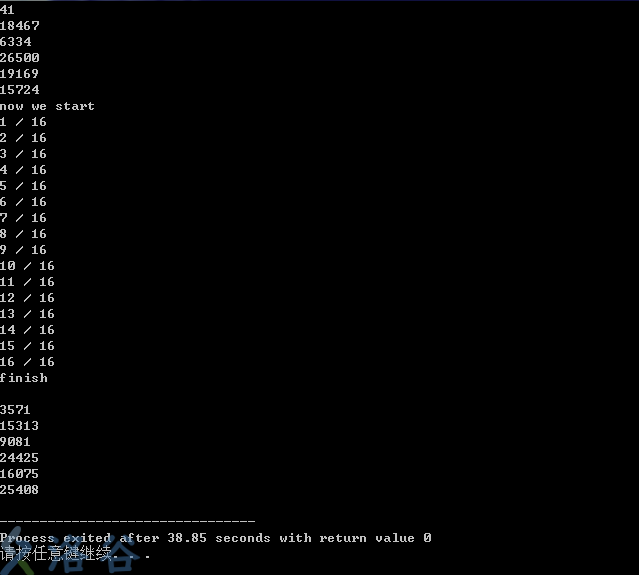（如果只想看实货的可以直接空降最后一个专题）

# 随机数定义及其性质

1. 它产生时后面那个数与前面的毫无关系

2. 给定样本的一部分和随机算法，无法推出样本的剩余部分

3. 其随机样本不可重现

continue->

# 伪随机数

### 有趣的小测试 ：

（ 根据网上的资料，rand函数循环节一般是2^32或2^64 ）

#include<iostream>
#include<stdlib.h>
#define MAXN 4294967296
using namespace std ;
int a ;
long long countn ;
int main ( ) {
countn = 0 ;
for( int i = 1 ; i <= 6 ; i ++ ){
a = rand ( ) ;
cout << a << endl ;
}
for( int i = 1 ; i <= 16 ; i ++ ){
countn ++ ;
cout << countn << " / 16 "<< endl ; // 用来跟踪进度的
for( int i = 6 ; i <= MAXN / 16 ; i ++ ){
a = rand ( ) ;
}
}
for( int i = 1 ; i <= 6 ; i ++ ){
a = rand ( ) ;
cout << a << endl ;
}
return 0 ;
}

（ 其中4294967296是2的32次方 ）（ 你们别问我为什么不去测试2^64次方，要测自己测，我没这个时间 ）

static unsigned long next = 1;

/* RAND_MAX assumed to be 32767 */
int myrand(void) {
next = next * 1103515245 + 12345;
return((unsigned)(next/65536) % 32768);
}

void mysrand(unsigned seed) {
next = seed;
}

（ 该代码摘自CSDN 原文链接

（ 所以我白测试了是吗 ）

# 真随机数

1. 它产生时后面那个数与前面的毫无关系

2. 给定样本的一部分和随机算法，无法推出样本的剩余部分

3. 其随机样本不可重现

（ 我咋就那么喜欢总结 ）

continue->

# 计算机的随机数

（ 本来想放点图可能可以增加可读性，但好像放不了什么... ）# 自然界的随机数

（ 说一下笔者的观点吧，经供参考。我认为世界上是有真随机存在的，不要问我为什么，唯心主义不需要解释 ）

# 随机数的应用

（ 感觉随机数好像也就这么点用，在密码学统计学中用的比较多 ）

# 如何生成优质随机数

### 来回摆动式

int f  = 3000 ;
int change = 0.999 ; // f和change是用来控制随机数幅度不断变小的
int con = -1 ;
int g = 1 ; // 控制正负交替
int newrand ( ) {
f *= change ;
g *= con ;
int tmp =  f * g * rand ( ) ;
return tmp ;
}

### 平均式

int p ; // 希望的分布位置
int newrand ( ) {
int tmp =  ( p + rand ( ) ) / 2 ; // 通过取于分布位置的平均数，是产生的数更加靠近希望分布
return tmp ;
}

### 多次调用不重复式

int count ;
int realrand ( ) {
count ++ ;
int t = clock ( ) + 1 ; // 使用当前时间
for ( int i = 1 ; i < 12121307 ; i ++ ) { // 降速（如果放到具体代码里面使用可以将此参数调低）
t += rand ( ) ;
}
t += clock ( ) ; // 降速后扩大时间变化
t *= -1234 ;
srand ( t * count + rand ( ) ) ; // 重置随机数种子
return rand ( ) ;
}

（@ =@）

（ > o < )